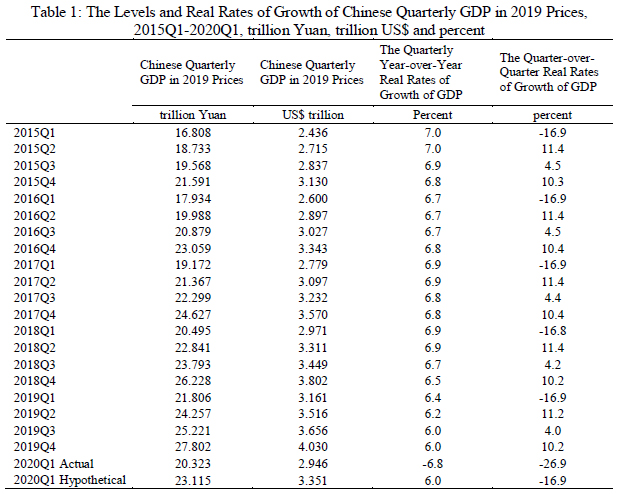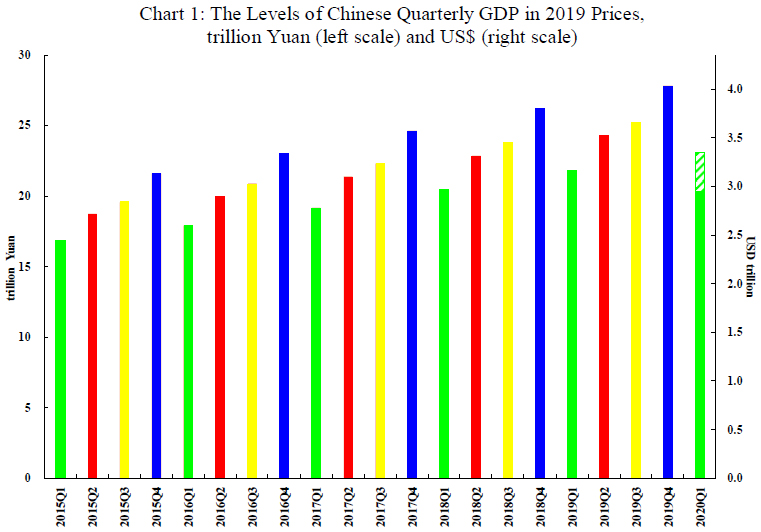Language : English 简体 繁體
Economy

# Projections of Possible Levels of Chinese GDP in 2020

Jun 10, 2020

In 2020Q1, there was an outbreak of the COVID-19 epidemic in China, which resulted in the temporary cessation of most economic activities in China due to the blockade of Hubei and Wuhan and lockdowns in many provinces and cities. Chinese real GDP declined by 6.8 percent, year-over-year, in the first quarter of 2020. A great deal of interest is focussed on the level of Chinese real GDP in 2020. The Chinese GDP in 2020 depends crucially on two developments—the speed of the economic recovery from the COVID-19 epidemic and the availability of additional economic stimulus.

In Table 1, the levels of Chinese quarterly GDP in 2019 prices since 2015Q1 are presented in both Yuan (column 2) and US\$ (column 3) at the average Yuan/US\$ exchange rate over 2019 of 6.9 Yuan per US\$. In addition, the quarterly year-over-year as well as the quarter-over-quarter real rates of growth are also presented in columns 4 and 5 respectively. We note, first of all, that the Chinese quarterly year-over-year real rates of growth have been declining gradually over the years. Moreover, in 2019, Chinese economic growth slowed down significantly because of the then rising China-U.S. trade war, and continues to be adversely affected by it. Table 1 shows that the quarterly year-over-year rate of growth declined gradually from 6.4% in 2019Q1 to 6.0% in 2019Q4. If there were no COVID-19 epidemic in China, the 2020Q1 GDP would probably have been approximately 6.0% higher than the 2019Q1 GDP of 21.81 trillion Yuan, or 23.12 (21.81 x 1.06) trillion Yuan. This would be our estimate of the hypothetical 2020Q1 GDP. The actual 2020Q1 GDP was 20.32 trillion Yuan. Thus, the loss of Chinese GDP due to the COVID-19 epidemic may be estimated as the difference between the hypothetical and the actual GDP, that is, 2.8 trillion Yuan (US\$405 billion), or 2.8 percent of the 2019 Chinese national GDP of 99.09 trillion Yuan.

However, a decline in Chinese GDP in the first quarter from the fourth quarter of the previous year is not unusual. An inspection of column 5 of Table 1 suggests that it occurs regularly, with an average non-annualised quarter-over-quarter decline of 16.9% between 2015 and 2019, but of course, not quite of the same magnitude as the decline of 26.9% actually realised in 2020Q1. Moreover, the first-quarter GDP typically accounts for less than 22 percent of the annual GDP, whereas the fourth-quarter GDP typically accounts for more than 28 percent of the annual GDP. This is due to the Spring Festival (lunar new year) holiday in China, which typically takes place between January and February, with all agricultural, manufacturing, mining and construction activities ceased.The levels of Chinese quarterly GDP in 2019 prices since 2015Q1 are also presented in Chart 1. For 2020Q1, we show in the same column both the actual level (in solid colour) and the hypothetical increase (in striped colour) of real GDP, with the hypothetical increase being our estimate of the loss in national GDP attributable to the COVID-19 epidemic, 2.8 trillion Yuan. Chart 1 clearly confirms the pronounced seasonality in the quarterly GDPs observed in Table 1. In particular, the rate of growth between Q1 and Q2 (from green column to red column) is much higher than the year-over-year growth rate of Q2 GDP (from red column to red column). In fact, the average rate of growth of GDP between Q1 and Q2 is 11.4% over the past five years (see Table 1, column 5).Thus, normally, 2020Q2 GDP is expected to increase by 11.4% from 2020Q1, so that the 2020Q2 GDP may be projected to be 22.64 (20.32 x 1.114) trillion Yuan. The 2020Q3 GDP is expected to increase by 4.3% from 2020Q2, to 23.61 trillion Yuan. The 2020Q4 GDP is expected to increase 10.3% to reach 26.04 trillion Yuan. For 2020 as a whole, by adding the actual 2020Q1 and the projected 2020Q2, Q3 and Q4 GDPs together, we obtain a projected annual GDP of 92.61 trillion Yuan. This represents a decline of 6.5% from the 2019 GDP of 99.09 trillion Yuan, which seems excessively low. Moreover, these projections are all based on the assumptions that there is no recovery effect and there will be no additional economic stimulus in China.

A major weakness in the above analysis is its failure to take into account that in 2020Q2, it is a recovery to a previously achieved level of output and not a fresh round of new growth, and hence should be more rapid. Consider an economy which has contracted by 50%, for whatever reason, if it is able to restore its output to just before the contraction, it will have grown 100% from the shrunken base, even though the contraction was only 50%. It is therefore not unreasonable to assume that GDP will be restored to at least the level of 2019Q4 in 2020Q2, once things return to normal. 2020Q1 GDP declined by 26.9% from 2019Q4. In order for 2020Q2 GDP to recover to the 2019Q4 level, it will have to grow at 36.8 (26.9/(1-0.269)) % quarter-over-quarter in 2020Q2. This is high, but not impossible, because it just represents recovery of lost grounds. With a 2020Q2 GDP of 27.80 (20.32 x 1.368) trillion Yuan, the 2020Q3 and Q4 GDPs may be projected successively as 29.00 (27.80 x 1.043) and 31.98 (29.00 x 1.103) trillion Yuan. This results in a projected GDP for the whole of 2020 of 109.1 trillion Yuan, or a rate of growth of 10.1% over 2019. However, a level of 2020Q2 GDP of 27.80 trillion Yuan implies a year-over-year rate of growth of the 2020Q2 GDP of 14.6%, which seems a little too high.

Still another possible alternative assumption is that the 2020Q2 GDP will recover to the same level as the 2019Q2 GDP of 24.26 trillion Yuan, a quarterly rate of growth of 19.4% instead of 36.8%. The 2020Q3 and Q4 GDPs can therefore be projected to reach 25.30 (24.26 x 1.043) and 27.90 (25.30 x 1.103) trillion Yuan respectively. These projections result in a projection of 2020 annual GDP of 97.78 trillion Yuan, implying a contraction of 1.4%.

Yet all the data on the re-opening and recovery on the Mainland to date suggest that the mostly likely outcome of 2020Q2 GDP is a year-over-year growth of 6.0%, that is, it would reach the level of 25.71 (24.26 x 1.06) trillion Yuan, implying a quarter-over-quarter rate of growth of 26.5%. The corresponding 2020Q3 and Q4 GDP’s may be projected successively as 26.82 (25.71 x 1.043) and 29.58 (26.82 x 1.103) trillion Yuan. This results in a projected GDP for the whole of 2020 of 102.43 trillion Yuan, or a rate of growth of 3.4% over 2019. However, all of these projections are still based on the assumption of no additional economic stimulus.

While a 10.1% annual rate of growth may appear too optimistic and a 1.4% contraction may appear too pessimistic, a 3.4% annual rate of growth for 2020 seems quite reasonable. However, all of these projections are predicated on the assumption of no new economic stimulus. If there were additional economic stimulus, the Mainland economy should be able to grow at higher rates in 2020Q3 and Q4. If we assume that with additional economic stimulus, the Mainland economy can grow at a rate 25% higher than the normal quarter-over-quarter rates of growth in 2020Q3 and Q4 respectively, it results in projections of 2020 quarterly GDPs of 27.09 (25.71 x 1.054) trillion Yuan and 30.58 (27.09 x 1.129) trillion Yuan respectively. On a year-over-year basis, the actual and our projected rates of growth of the four quarters in 2020 are -6.8%, 6.0%, 7.4% and 10.0% respectively.

If we now sum up the actual and these projected levels of quarterly GDPs for 2020, we obtain a projection of 2020 real GDP of 103.70 trillion Yuan, or an increase of 4.65% from 2019, with additional economic stimulus. However, without any economic stimulus, the rate of growth of GDP for 2020 is likely to be around 3.4%, as we have seen above. If, in the meantime, the rest of the global economy also recovers in 2020Q3 and 2020Q4, the Chinese rates of growth would be even higher, perhaps even exceeding 5% for 2020 as a whole, with additional economic stimulus. Of course, it remains to be seen how fast the Mainland economy will be able to recover for the rest of 2020. Our assumption and expectation is that the rates of growth for the remaining three quarters of 2020 will be significantly above normal.

 This is the average rate of growth between the GDPs of Q1 and Q2 from 2015-2019.

 This is the average rate of growth between the GDPs of Q2 and Q3 from 2015-2019.

 This is the average rate of growth between the GDPs of Q3 and Q4 from 2015-2019.

 In fact, the value-added of industry grew 33% month-to-month in March 2020.

 The International Monetary Fund has projected Chinese GDP to grow at 1.2% in 2020.  The World Bank has projected Chinese GDP to grow at 1% in 2020 in June 2020.

You might also like